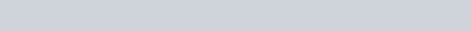Evaluate the following integrals:
Question:

Evaluate the following integrals:

$\int \cos ^{-1}\left(4 x^{3}-3 x\right) d x$

Solution: